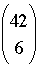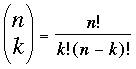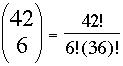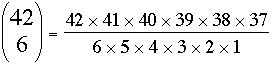SEARCH HOMEMath Central Quandaries & QueriesQuestion from Marcus: If i have 42 numbers to choose from and i could only choose 6 numbers at a time without choosing the same number twice in the same row, how many possibilities are there?Hi Marcus,

The number of ways of choosing 6 objects from 42 objects is called 42 choose 6 and is written by mathematicians asbut sometimes you see it designated 42C6. The definition iswhere k! = k × (k-1) × (k-2) × (k-3) × ··· × 1. (For example 5! = 5 × 4 × 3 × 2 × 1 = 120.) ThusAfter some simplification this becomesI'll let you do the arithmetic.

PennyMath Central is supported by the University of Regina and The Pacific Institute for the Mathematical Sciences.